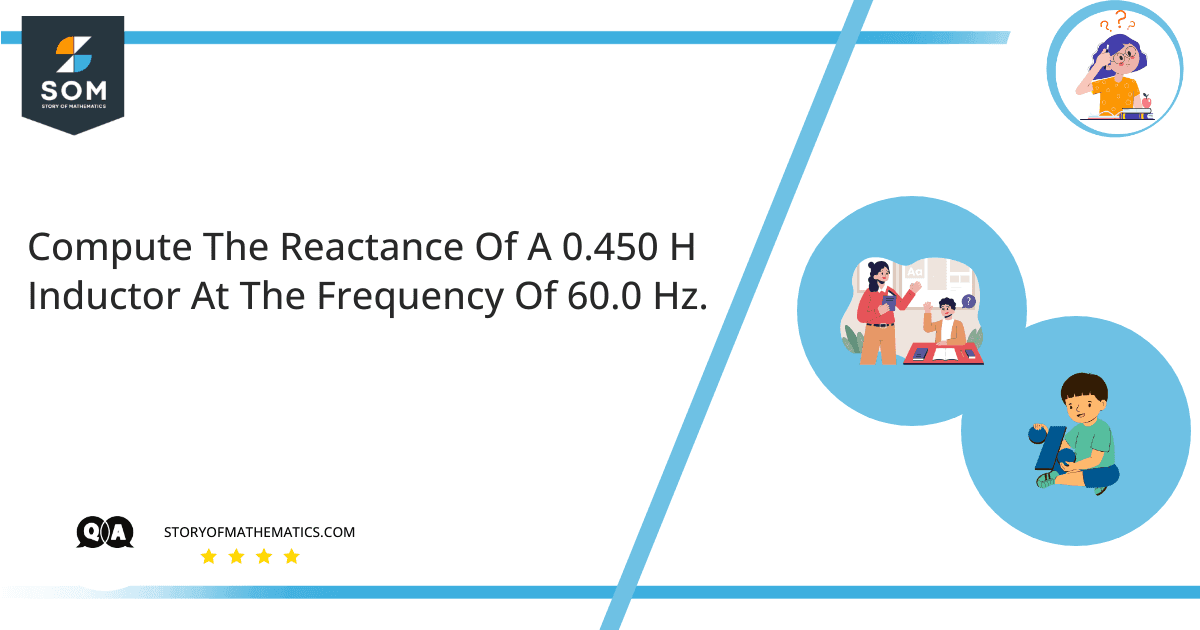# Compute the reactance of a 0.450 H inductor at the frequency of 60.0 Hz. Compute the reactance of a 2.50 microfarad capacitor at the same frequencies.The aim of this question is to develop an understanding of the reactance of capacitors and inductors. It also covers the concept of the resonance frequency.

The reactance of an inductor against the flow of alternating current can be computed using the following formula:

$X_{ L } \ = \ \omega \ L$

The reactance of a capacitor against the flow of alternating current can be computed using the following formula:

$X_{ C } \ = \ \dfrac{ 1 }{ \omega \ C }$

In above equations, $X$ represents the reactance, $\omega$ is the frequency in $rad/sec$, $L$ is the inductance, and $C$ is the capacitance.

The resonance frequency is such a frequency where the capacitive reactance due to the capacitors and inductive reactance due to the inductance becomes equal in magnitude for a given circuit. Mathematically:

$X_{ L } \ = \ X_{ C }$

Part (a) – The reactance of an inductor against the flow of alternating current can be computed using the following formula:

$X_{ L } \ = \ \omega \ L$

Since:

$\omega \ =\ 2 \pi f$

So the above equation becomes:

$X_{ L } \ = \ 2 \pi f \ L$

Given:

$f \ = \ 60 \ Hz$

$L \ = \ 0.45 \ H$

Substituting these values in the above equation:

$X_{ L } \ = \ 2 \pi ( 60 ) \ ( 0.45 )$

$\Rightarrow X_{ L } \ = \ 169.65 \ \Omega$

Part (b) – The reactance of a capacitor against the flow of alternating current can be computed using the following formula:

$X_{ C } \ = \ \dfrac{ 1 }{ \omega \ C }$

Since:

$\omega \ =\ 2 \pi f$

So the above equation becomes:

$X_{ C } \ = \ \dfrac{ 1 }{ 2 \pi f \ C }$

Given:

$f \ = \ 60 \ Hz$

$L \ = \ 2.5 \ \mu F$

Substituting these values in the above equation:

$X_{ C } \ = \ \dfrac{ 1 }{ 2 \pi ( 60 ) \ ( 2.5 \mu ) }$

$\Rightarrow X_{ C } \ = \ \dfrac{ 1 }{ 942.48 \ \mu }$

$\Rightarrow X_{ C } \ = \ 1061.03 \ \Omega$

## Numerical Results

$\Rightarrow X_{ L } \ = \ 169.65 \ \Omega$

$\Rightarrow X_{ C } \ = \ 1061.03 \ \Omega$

## Example

In the above question, find the frequency where the reactance of both the inductor and capacitor becomes equal.

Given:

$X_{ L } \ = \ X_{ C }$

$2 \pi f \ L \ = \ \dfrac{ 1 }{ 2 \pi f \ C }$

$f^{ 2 } \ = \ \dfrac{ 1 }{ 4 \pi^{ 2 } \ L \ C }$

$f \ = \ \dfrac{ 1 }{ 2 \pi \ \sqrt{ L \ C } }$

Substituting values:

$f \ = \ \dfrac{ 1 }{ 2 \pi \ \sqrt{ ( 0.450 ) \ ( 2.5 \ \mu ) } }$

$f \ = \ \dfrac{ 1 }{ 2 \pi \ ( 1.06 \ mili ) }$

$f \ = \ \dfrac{ 1 }{ 6.664 \ mili ) }$

$f \ = \ 150 \ Hz$